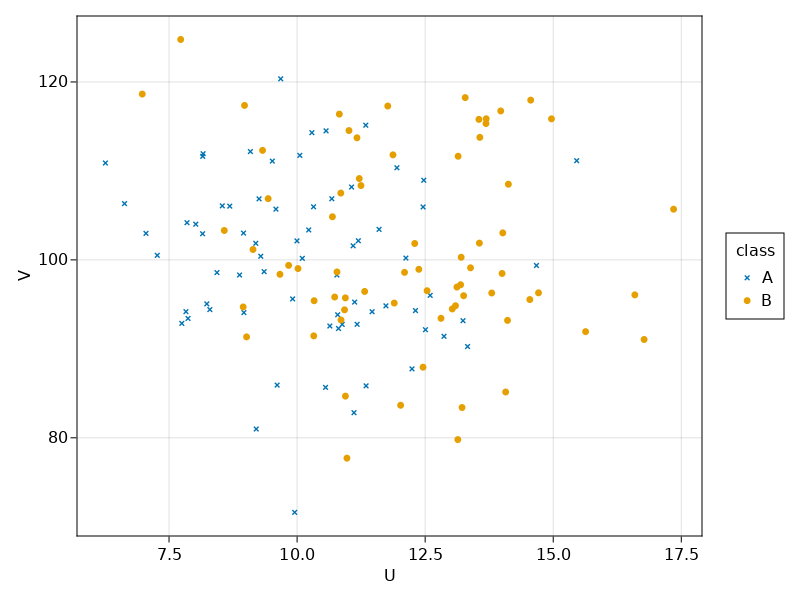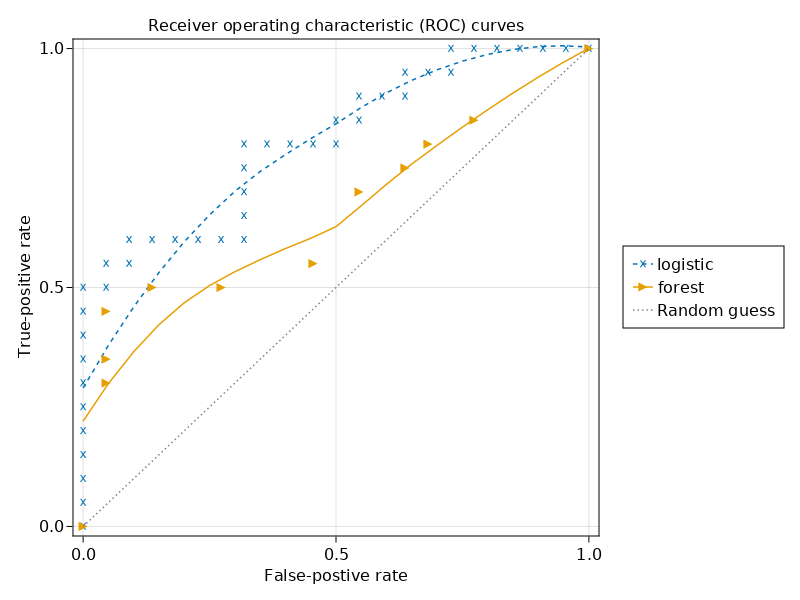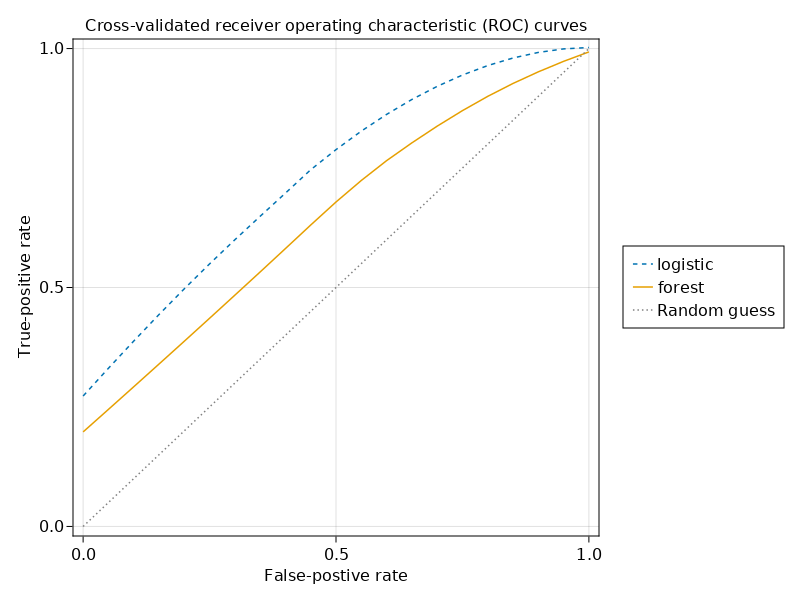Below is example code for fitting and evaluating a linear regression and random forest classifier in Julia. I've used both models to have a baseline for the random forest. The model is evaluated on a mock variable $U$ generated from two distributions, namely

\begin{aligned} d_1 &= \text{Normal}(10, 2) \: \: \text{and} \\ d_2 &= \text{Normal}(12, 2), \end{aligned}

The random variable $V$ is just noise meant to test the classifier, generated via

$$V \sim \text{Normal}(100, 10)$$

This data isn't meant to show that random forests are good classifiers per se. One way to do that would be to have about the same or more variables than observations (Biau & Scornet, 2016).

## Data generation

begin
import MLJGLMInterface
import MLJDecisionTreeInterface

using CairoMakie
using CategoricalArrays
using DataFrames
using Distributions
using Loess: Loess
using MLJBase
using MLJ
using StableRNGs: StableRNG
using Random
end
classlabels = ["A", "B"];
df = let
# Number of elements per class.
n = 70
μ1 = 10
μ2 = 12
σ = 2

d1 = Normal(μ1, σ)
d2 = Normal(μ2, σ)

Random.seed!(12)
classes = repeat(classlabels, n)

df = DataFrame(
class = categorical(classes),
U = [class == "A" ? rand(d1) : rand(d2) for class in classes],
V = rand(Normal(100, 10), 2n)
)
end
class U V
"A" 10.6777 106.869
"B" 14.0715 85.1292
"A" 7.0505 102.981
"B" 17.3497 105.68
"A" 8.29689 94.4151
"B" 11.2469 108.338
"A" 9.91297 95.6106
"B" 15.6322 91.9133
"A" 8.9616 94.0726
"B" 13.6919 115.841
...
"B" 13.22 83.3854
X = (; df.U, df.V);
y = df.class;

If we plot this, we can see that the points of "B" are higher on average for U. In other words, the points lie more on the right:

let
fig = Figure()
ax = Axis(fig[1, 1]; xlabel="U", ylabel="V")
classmarkers = [:xcross, :circle]
for (label, marker) in zip(classlabels, classmarkers)
filtered = filter(:class => ==(label), df)
scatter!(ax, filtered.U, filtered.V; label, marker)
end
Legend(fig[1, 2], ax, "class")
fig
end## Train and test split

Let's split our data before continuing. Training and evaluating (testing) on the same data is not great because we want to know how well our model generalizes. It is easy to make correct predictions when you have seen the data which you need to predict already. For more information, see topics such as overfitting. So, to avoid this problem, we split the data up in a train and test set.

train, test = let
rng = StableRNG(123)
MLJ.partition(eachindex(df.class), 0.7; shuffle=true, rng)
end;

## Model fitting

Now, we can fit a model in order to determine the accuracy later:

logistic = let
LinearBinary = @load LinearBinaryClassifier pkg=GLM verbosity=0
LinearBinary()
end;
fitted_logistic = let
Random.seed!(11)
mach = machine(logistic, X, y)
fit!(mach; rows=train)
mach
end;
r2(x) = round(x; digits=2);
coefficients = r2.(fitted_params(fitted_logistic).coef)
2-element Vector{Float64}:
0.44
0.03

The second coefficient in the linear model is close to zero. This is exactly what the model should do since V is random noise.

Let's also fit the random forest model:

forest = let
Random.seed!(11)
DecisionTree = @load DecisionTreeClassifier pkg=DecisionTree verbosity=0
tree = DecisionTree()
EnsembleModel(tree; n=10)
end;

## Accuracy

Now that we know how to fit the models and verified the linear model, we can compare the accuracies and plot the receiver operating characteristic (ROC) curves. In this curve, higher means a better predictive performance.

Here, I've used Makie.jl instead of AlgebraOfGraphics.jl. This is more barebones, that is, I had to write code to smooth the line. This took a bit of extra time upfront, but allows for much greater flexibility which saves time in the long run.

function smooth(xs, ys)
model = Loess.loess(xs, ys; span=1.0)
us = range(extrema(xs)...; step=0.05)
vs = Loess.predict(model, us)
return us, vs
end;

The next function fits a model and obtains the false-positive rates fprs and the true-postive rates tprs:

function fprs_tprs(model, X, y, train, test)
mach = machine(model, X, y)
fit!(mach; rows=train)
predictions = MLJ.predict(mach; rows=test)
fprs, tprs, _ = roc_curve(predictions, df.class[test])
return fprs, tprs
end;

For plotting, I'm first defining a struct here. Objects of this RocCurve type can be sent to the plotting function in order to show multiple curves in one plot. Defining a struct like this is useful because it makes things more explicit. We can enforce that each field has the correct type, which is not possible for things like NamedTuples.

begin
struct RocCurve
fprs::Vector{Float64}
tprs::Vector{Float64}
label::String
linestyle::Union{Nothing,Symbol}
marker::Symbol
end

function RocCurve(model::MLJ.Model, label::String, linestyle, marker)
# Using the train-test split defined above.
fprs, tprs = fprs_tprs(model, X, y, train, test)
return RocCurve(fprs, tprs, label, linestyle, marker)
end
end;
curves = [
RocCurve(logistic, "logistic", :dash, :x),
RocCurve(forest, "forest", nothing, :rtriangle)
];
function plot_curves(
curves::Vector{RocCurve};
xlabel="False-postive rate",
ylabel="True-positive rate",
show_points::Bool=true
)
fig = Figure()
ax = Axis(fig[1, 1]; title, xlabel, ylabel)

plotted = map(curves) do curve
fprs = curve.fprs
tprs = curve.tprs
line = lines!(ax, smooth(fprs, tprs)...; curve.linestyle)
objects = []
if show_points
scat = scatter!(ax, fprs, tprs; markersize=12, curve.marker)
objects = [scat, line]
else
objects = [line]
end
(; objects, curve.label)
end
random = lines!(ax, 0:1, 0:1; color=:gray, linestyle=:dot)
push!(plotted, (; objects=[random], label="Random guess"))
ylims!(ax, -0.02, 1.02)
xlims!(ax, -0.02, 1.02)

Legend(
fig[1, 2],
getproperty.(plotted, :objects),
getproperty.(plotted, :label)
)
fig
end;
plot_curves(curves)From this plot, we can learn that the logistic model has a higher area under the curve meaning that it is a better predictive model on our single train test split. This makes sense because the random forest is very likely to overfit our data, that it, it will fit patterns which hold for the test set but do not necessarily hold in general.

However, it could be that the conclusion would be different if the train test split was slightly different. To draw more robust conclusions, we need cross-validation.

## K-fold cross-validation

By doing a train and test split, we basically threw a part of the data away. For small datasets, like the dataset in this example, that is not very efficient. Therefore, we also do a k-fold cross-validation. This has the benefit that we use all the data that we have for evaluations. We can evaluate the model via the evaluate function from MLJ.jl:

function evaluate_model(model)
rng = StableRNG(8)
resampling = CV(; nfolds=10, shuffle=true, rng)
evaluations = evaluate(model, X, y; resampling, measure=auc, verbosity=0)
average = r2(only(evaluations.measurement))
per_fold = r2.(only(evaluations.per_fold))
return (; average, per_fold)
end;
evaluate_model(logistic)
(average = 0.76,
per_fold = [0.71, 0.83, 0.96, 0.75, 0.65, 0.62, 0.9, 0.82, 0.91, 0.49],)
evaluate_model(forest)
(average = 0.7,
per_fold = [0.58, 0.69, 0.92, 0.52, 0.73, 0.73, 0.73, 0.69, 0.67, 0.78],)

So, our previous conclusion still holds because the average is higher for the logistic model. Still, it would be nice to see plots instead of numbers. Let's make them.

## ROC curves for k-fold cross-validation

In scikit-learn, there is a function to create roc plots with cross-validation. Well, there is good news and bad news. The bad news is, scikit-learn is a Python library and making Julia and Python work together is difficult to set up if you want to run your code locally and in CI jobs. The good news is, thanks to Pluto.jl, MLJ.jl and Makie.jl, it shouldn't be too hard to make such a plot ourselves in pure Julia.

The MLJ.jl API only returns the AUC scores per fold and not the number of true and false-positives. We need those to be able to plot the ROC curves. To work around this, we can pull the train-test rows from evaluations. Next, we have to fit the models on those train-test rows, get the true and false-positives and plot them:

function cross_validated_fprs_tprs(model)
rng = StableRNG(6)
resampling = CV(; nfolds=10, shuffle=true, rng)
Random.seed!(12)
evaluations = evaluate(model, X, y; resampling, measure=auc, verbosity=0)
return map(evaluations.train_test_rows) do (train, test)
fprs_tprs(model, X, y, train, test)
end
end;

To combine the results of the multiple cross-validation runs, I've put all the false and true-positive rates in one big vector:

function combine_cross_validations(model)
multiple_fprs_tprs = cross_validated_fprs_tprs(model)
fprs = vcat(first.(multiple_fprs_tprs)...)
tprs = vcat(last.(multiple_fprs_tprs)...)
return fprs, tprs
end;

It would be nicer to use the mean of each of the n runs in each cross-validation split. Unfortunately that was impossible for the random forest for which n was not constant over the splits. I suspect that the difference is caused by the forest model being unable to fit on some splits.

We can convert these combined fprs and tprs to RocCurves and plot them:

function cross_validation_curve(model, label::String, linestyle, marker)
fprs, tprs = combine_cross_validations(model)
return RocCurve(fprs, tprs, label, linestyle, marker)
end;
cv_curves = [
cross_validation_curve(logistic, "logistic", :dash, :x);
cross_validation_curve(forest, "forest", nothing, :rtriangle)
];
let
title = "Cross-validated receiver operating characteristic (ROC) curves"
plot_curves(cv_curves; title, show_points=false)
endNoice! The result remains the same as before, namely the area under the curve is higher for the logistic model. Hence, according to the k-fold cross-validation, the logistic model has a better predictive performance.

What does worry me a bit though is how the lines do not move nicely through the points (0.0, 0.0) and (1.0, 1.0). This can be explained by the fact that we used an average on a relatively small sample. With bigger samples, the outcomes are more robust and the lines will look better. Also, the difference between the random forest and the logistic models will become smaller. To see this, you can open this notebook via the link below, change the n at the top and see how the plots change.

## Appendix

Built with Julia 1.7.2 and

CairoMakie 0.7.5
CategoricalArrays 0.10.5
DataFrames 1.3.3
Distributions 0.25.53
Loess 0.5.4
MLJ 0.18.2
MLJBase 0.20.1
MLJDecisionTreeInterface 0.2.2
MLJGLMInterface 0.3.0
StableRNGs 1.0.0